### Home > MC1 > Chapter 6 > Lesson 6.3.1 > Problem6-122

6-122.
1. . Multiply the following fractions. Homework Help ✎

1.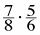2.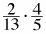3.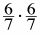4.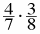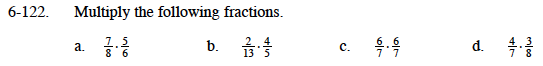Refer to the Math Notes box below if you are having trouble getting started.

Multiply the numerators together and the denominators together.

$\left ( \frac{7}{8} \right )\left ( \frac{5}{6} \right )=\frac{(7)(5)}{(8)(6)}$

Now, simplify the fraction.

$\frac{35}{48}$

Follow the steps from part (a) and refer to the Math Notes box if you are having trouble.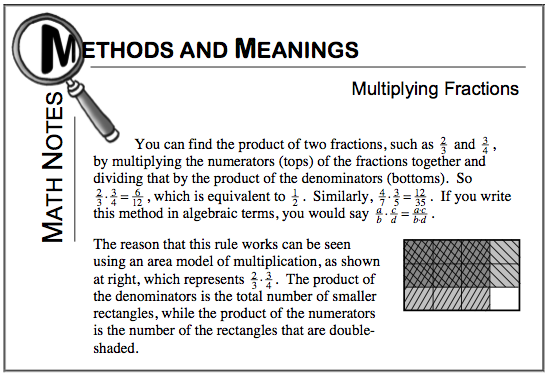$\frac{36}{49}$